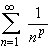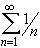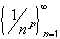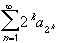#Interactive Real Analysis

Next | Previous | Glossary | Map

## 4.3. Special Series

### Theorem 4.2.11: p Series

The seriesis called a p Series.
• if p > 1 the p-series converges
• if p1 the p-series diverges

 Examples 4.2.12:Does the seriesconverge or diverge ?Does the seriesconverge or diverge ?Does the seriesconverge or diverge ? (This is the same series as in the example for the Limit Comparison test . Are we running in a circle here ?)
Proof:

If p < 0 then the sequenceconverges to infinity. Hence, the series diverges by the Divergence Test.

If p > 0 then consider the series=The right hand series is now a Geometric Series.
• if 0 < p1 then 2 1-p1, hence the right-hand series diverges
• if 1 < p then 2 1-p < 1, hence the right-hand series converges

Now the result follows from the Cauchy Condensation test .

Next | Previous | Glossary | Map